﻿ 基于自然邻居邻域图的无参数离群检测算法
«上一篇文章快速检索 高级检索

 智能系统学报2019, Vol. 14Issue (5): 998-1006  DOI: 10.11992/tis.2018090320

### 引用本文FENG Ji, RAN Ruisheng, WEI Yan. A parameter-free outlier detection algorithm based on natural neighborhood graph[J]. CAAI Transactions on Intelligent Systems, 2019, 14(5): 998-1006. DOI: 10.11992/tis.201809032.### 文章历史

A parameter-free outlier detection algorithm based on natural neighborhood graph
FENG Ji, RAN Ruisheng , WEI Yan
College of Computer and Information Science, Chongqing Normal University, Chongqing 401331, China
Abstract: This study aims to deal with the practical shortages of nearest-neighbor-based data mining techniques, particularly outlier detection. In particular, when data sets have arbitrarily shaped clusters and varying density, determining the appropriate parameters without a priori knowledge becomes difficult. To address this issue, on the basis of the natural neighbor method, which can better reflect the relationship between elements in a data set than the k-nearest neighbor method, we present a graph called the weighted natural neighborhood graph for outlier detection. The weighted natural neighborhood graph does not need to set parameters artificially in the entire process and can identify global and local outliers in the data set with different distribution characteristics. The outlier detection results of artificial dataset and real data prove that the algorithm can obtain an effect similar to that of the optimal parameter in the algorithm with parameters. The algorithm detection result is far better than that of most parameter-sensitive algorithms and is much better than that of the parameter-insensitive algorithm, which has stronger universality and more practicality.
Key words: parameter-free    adaptive neighbor    nearest neighbor    weighted graph    outlier detection    outlier factor    global outlier    local outlier

1 传统离群检测算法

Ha等利用不稳定因子提出了一种新的对参数不敏感的离群检测算法INS(instability factor)。INS改善了KNN算法中离群检测算法对参数敏感的问题，使得离群检测的准确率能够在较大的范围内不会随着参数的改变而产生很大的变化，且可以检测出数据集中的全局和局部离群点。但是INS算法对参数的不敏感性牺牲了一部分离群检测的准确率，即INS的离群检测结果趋于稳定时检测准确率往往低于邻域参数选择合理时的其他算法。而且INS算法很难同时检测出局部离群点和全局离群点，检测局部离群点时需要调整不稳定因子的设定。

2 基于自然邻居思想的离群检测算法 2.1 自然邻居概述

 $\begin{gathered} (\forall {x_i})(\exists {x_j})(r \in N) \wedge ({x_i} \ne {x_j}) \\ \to ({x_i} \in {\rm{KNN}}_{r}({x_j})) \wedge ({x_j} \in {\rm{KNN}}_{r}({x_i})) \\ \end{gathered}$

 ${x_i} \in NN({x_j}) \Leftrightarrow ({x_i} \in {\rm{KNN}}_{\lambda }({x_j})) \wedge ({x_j} \in {\rm{KNN}}_{\lambda }({x_i}))$

 $\begin{gathered} {\textit{λ}} \buildrel \Delta \over ={r_{r \in N}}\{ r|(\forall {x_i})(\exists {x_j})(r \in N) \wedge ({x_i} \ne {x_j}) \\ \to ({x_i} \in {\rm{KNN}}_{r}({x_j})) \wedge ({x_j} \in {\rm{KNN}}_{r}({x_i}))\} \\ \end{gathered}$

 $e({v_i},{v_j}) \in E \Leftrightarrow {x_j} \in {\rm{NN}}({x_i})$

2.2 基于加权自然邻居邻域图的离群检测算法

 $w({x_i},{x_j}) = \\ \min (r)\{ r|({x_i} \in {\rm{KNN}}_{r}({x_j})) \wedge ({x_j} \in {\rm{KNN}}_{r}({x_i}))\}$

 $f(p) = \frac{{\mathop {\max }\limits_{q \in Q} w(p,q) \mathop {\min }\limits_{q \in Q} w(p,q)}}{{{\rm{dgree}}(p)}}$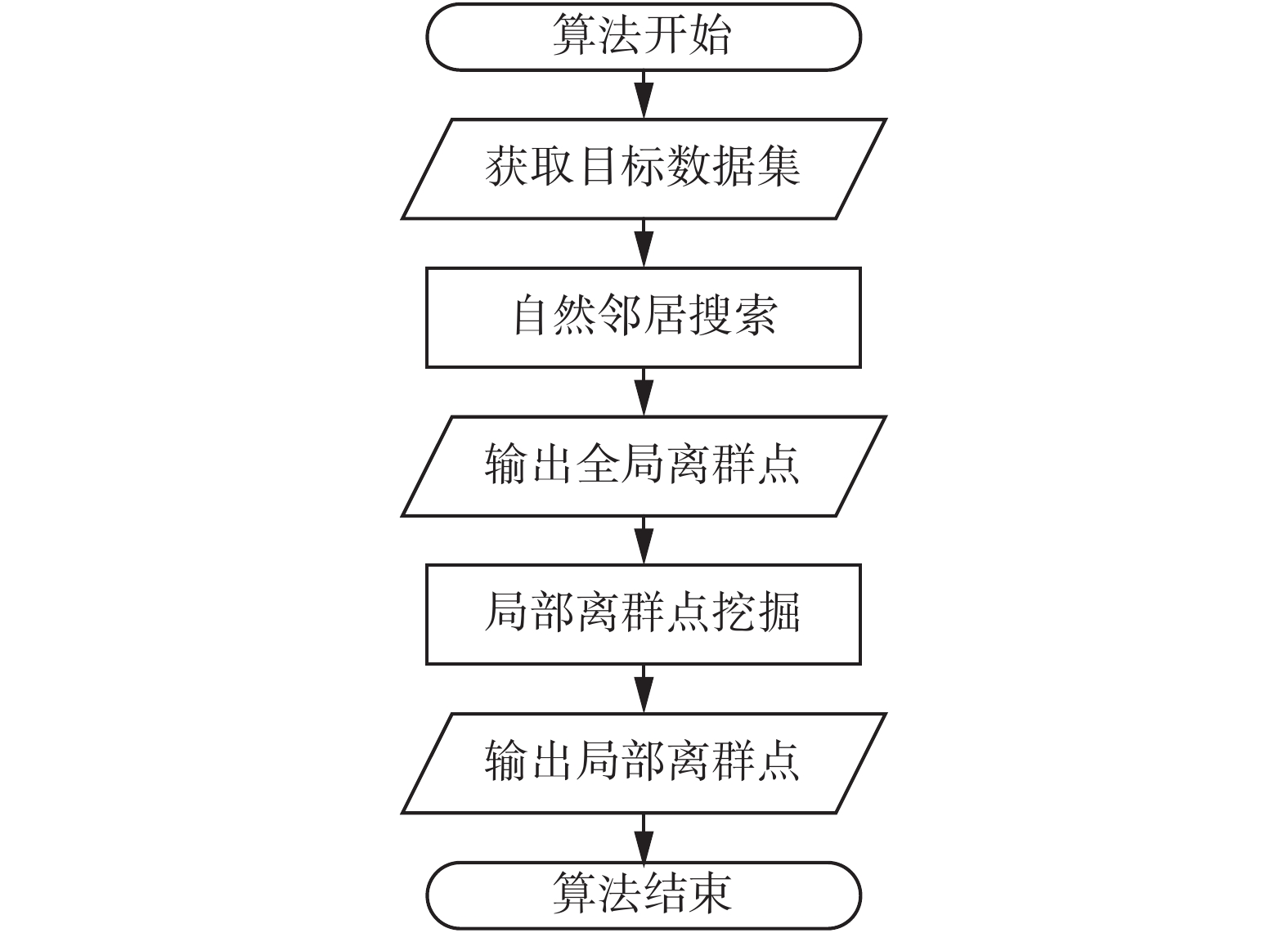Download: 图 1 基于加权自然邻居邻域图的离群检测算法流程图 Fig. 1 Flowchart of the WNaNG outlier detection algorithm

1) 初始化k=0，并创建数据集X对应的k-d树T

2) 令k=k+1，并利用k-d树T找到数据集中每个点的k最近邻居；

3) 分析当前的邻居关系，将互为k最近邻居的两点构成一条边，并记录当前的k作为该边的权值；

4) 重复执行步骤2)~3)，直到数据集X中所有点都至少具有一个邻居，或连续r次未增加新的边，其中 $r = \sqrt {k - r}$

5) 将加权自然邻居邻域图中没有边的点标记为全局离群点，并计算出剩余的局部离群点个数nl

6) 根据当前所有已知边的信息构造加权自然邻居邻域图。

1) 对邻域图进行遍历，找到每个点的所有加权边；

2) 根据定义6计算所有点的自然离群因子f

3) 对所有点的自然离群因子进行降序排序，前nl个点即为局部离群点。

2.3 自然邻居离群因子离散图分析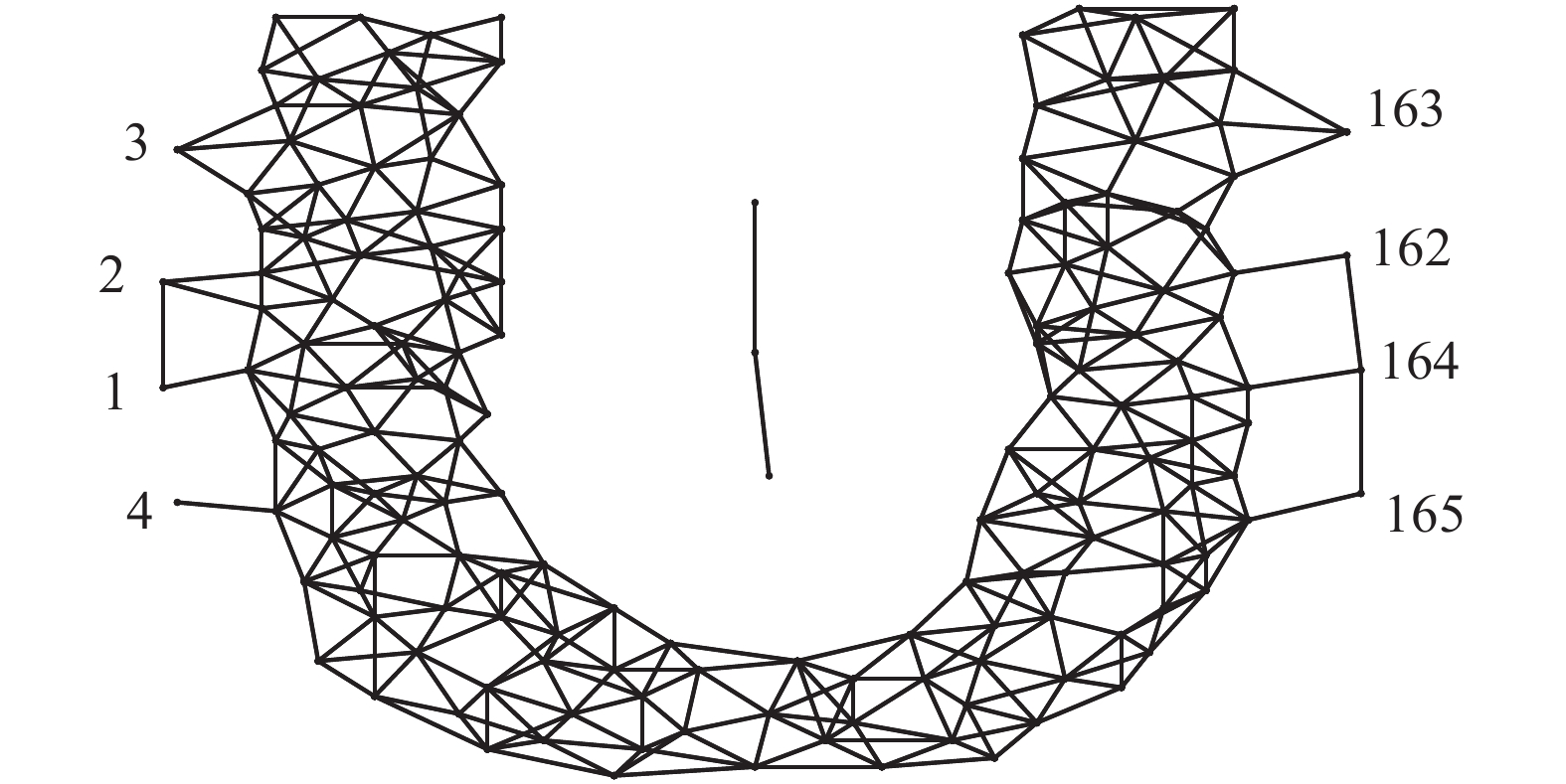Download: 图 2 自然邻居邻域图和局部离群点示意 Fig. 2 Natural neighbor graph and local outliers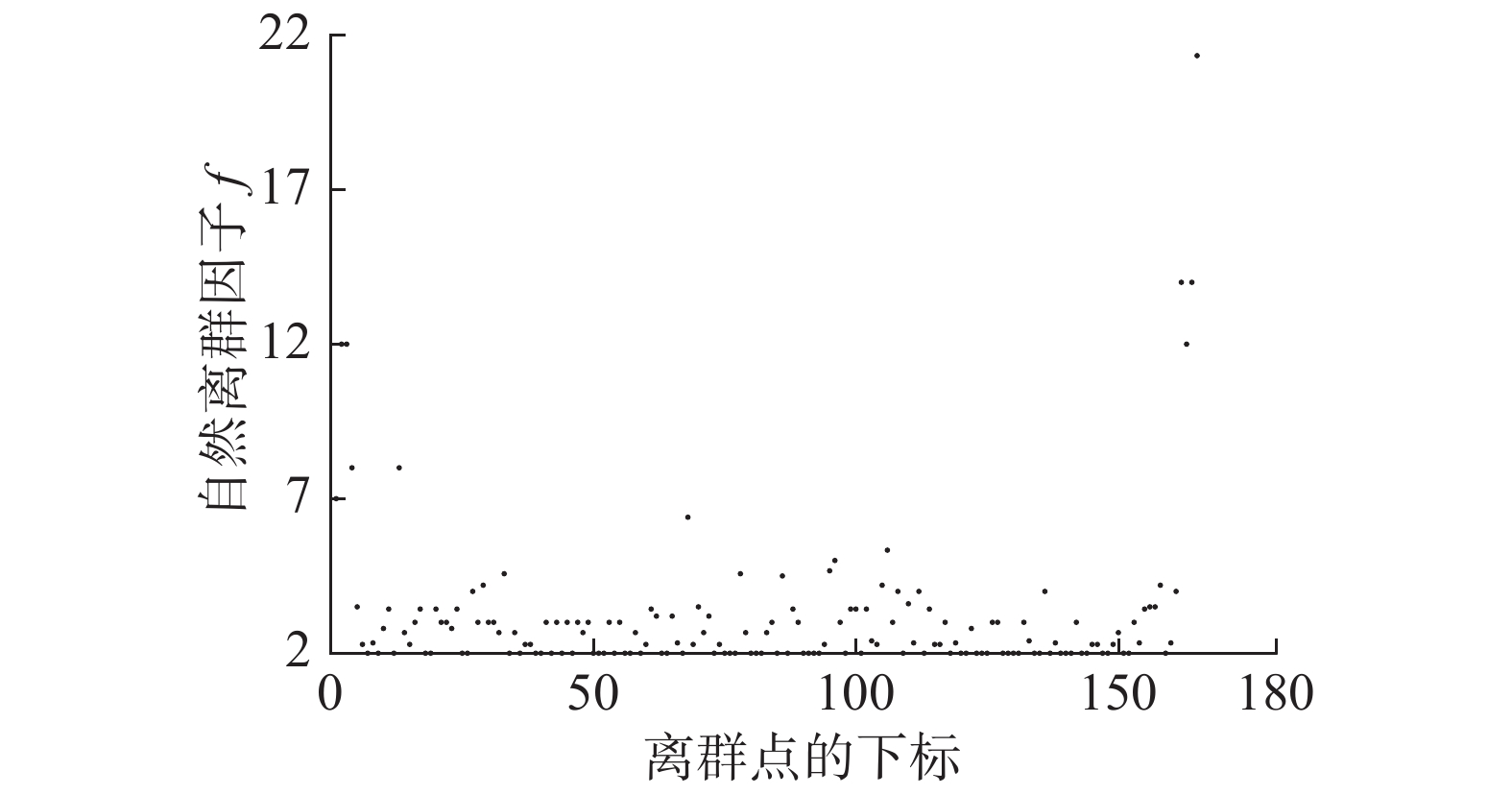Download: 图 3 数据集中各个数据点对应的自然邻居离群因子 Fig. 3 Natural neighbor outlier factor of each data point

2.4 算法复杂度分析

3 实验结果 3.1 人工数据集实验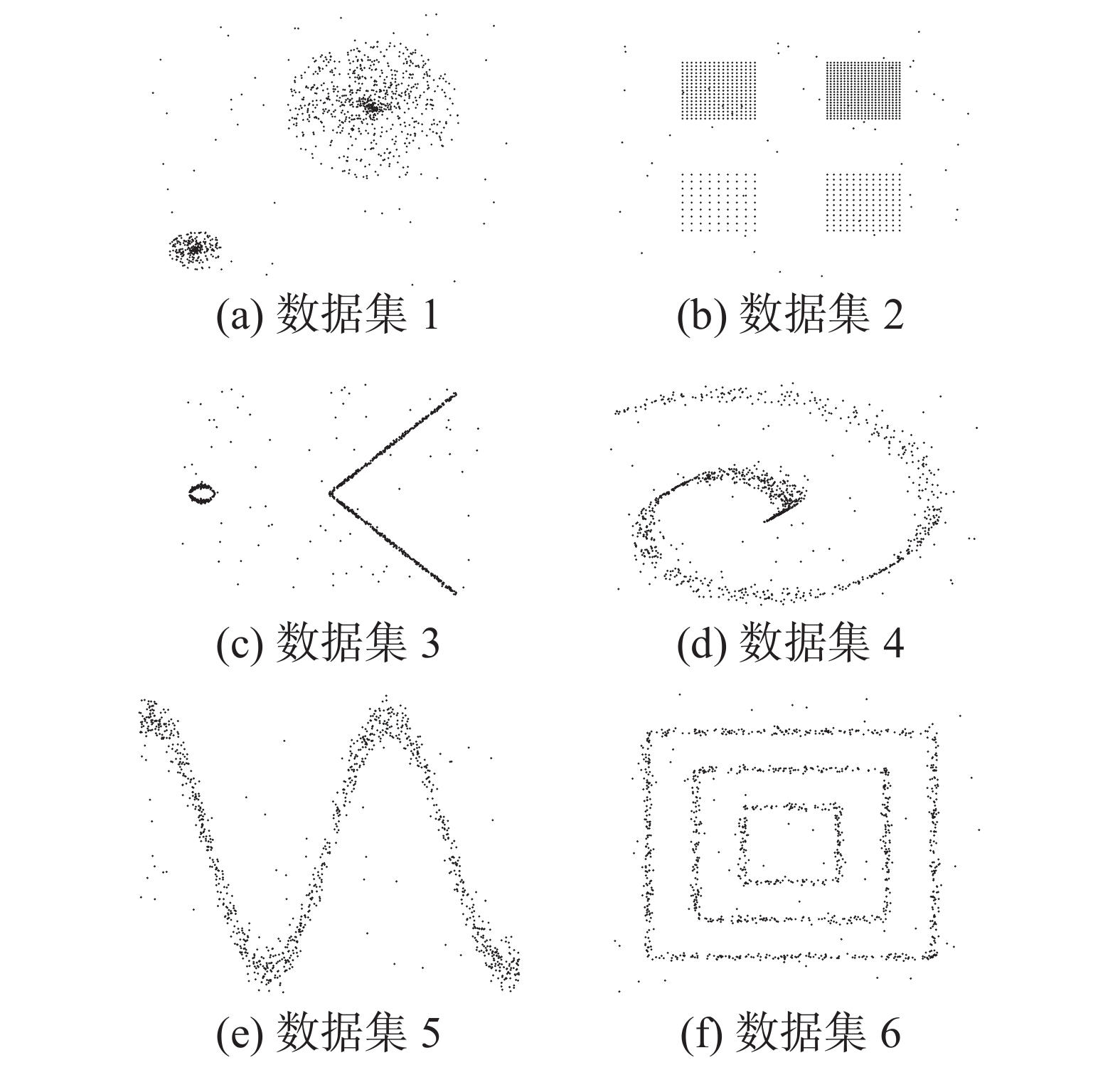Download: 图 4 包含离群点的6个人工数据集 Fig. 4 Six synthetic data sets of the outliers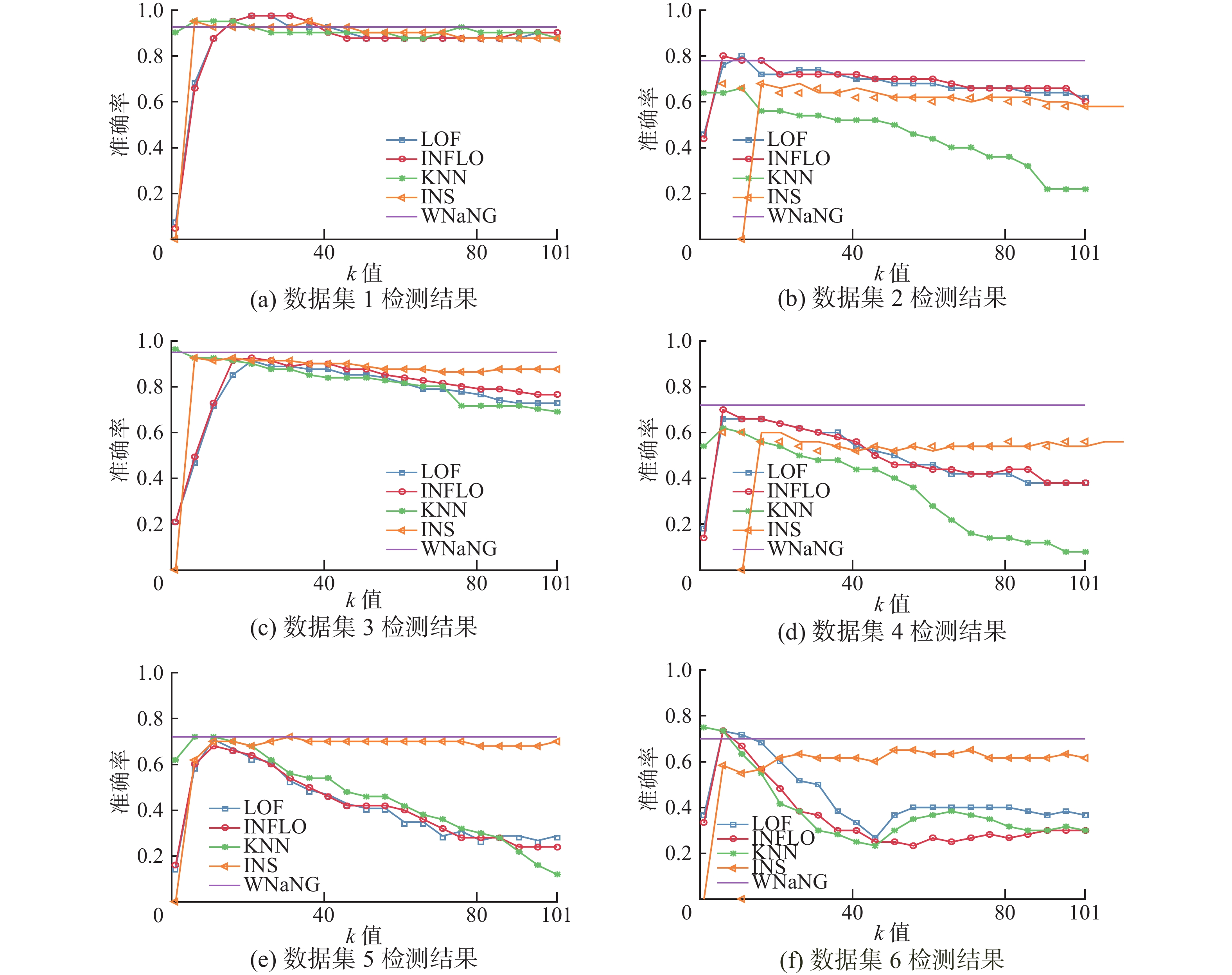Download: 图 5 5种离群检测算法在取不同k值时离群检测准确率对比 Fig. 5 Outlier detection accuracies of five detection methods over a range of k values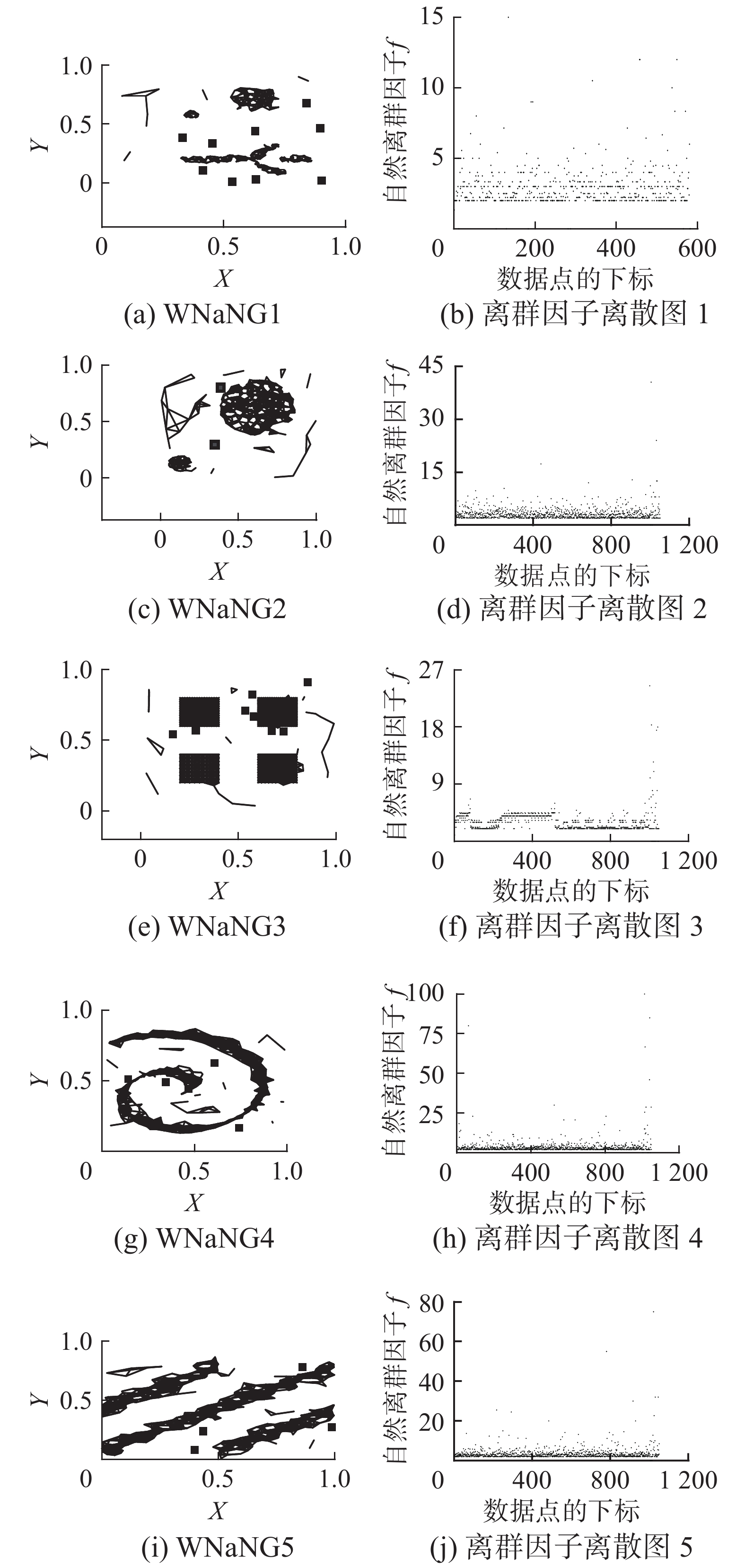Download: 图 6 局部离群点与自然邻居离群因子离散图 Fig. 6 Local outliers and NaNOF scatter
3.2 真实数据集实验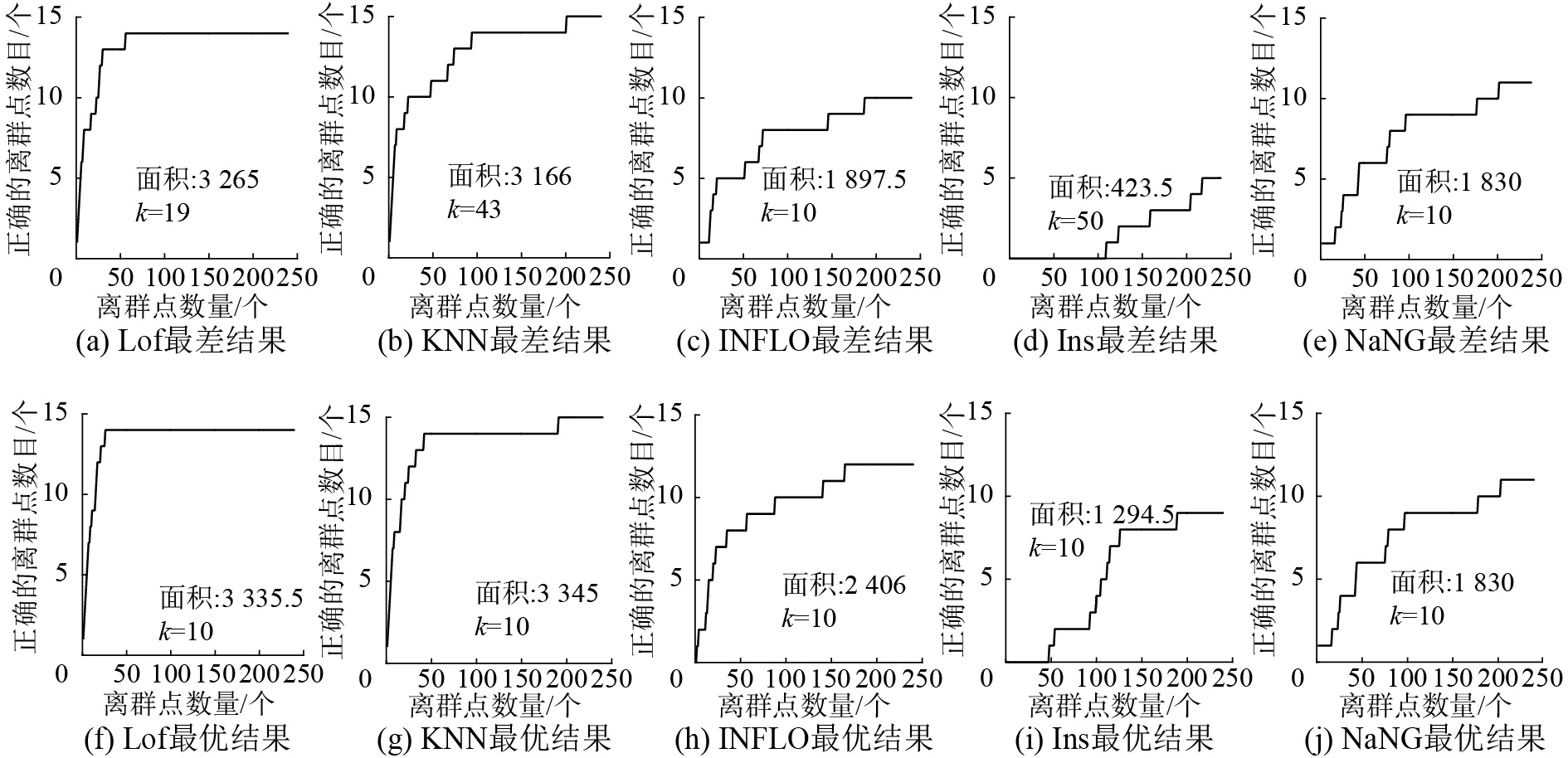Download: 图 7 CANCER数据集的ROC曲线下面积 Fig. 7 Area under the ROC curves of CANCER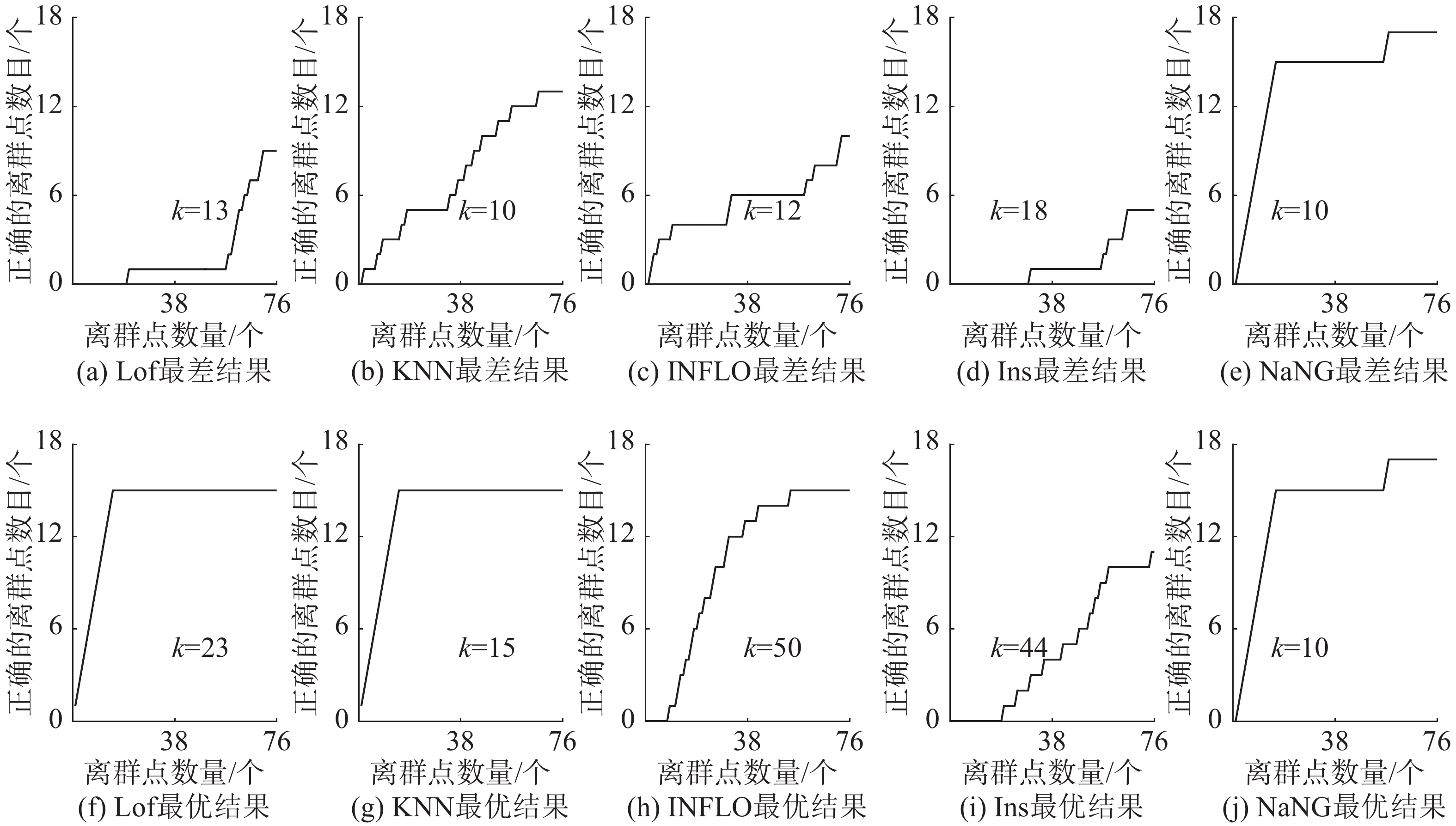Download: 图 8 IRIS数据集的ROC曲线下面积 Fig. 8 Area under the ROC curves of IRIS

4 结束语

  BOLTON R J, HAND D J. Statistical fraud detection: a review[J]. Statistical science, 2002, 17(3): 235-255. DOI:10.1214/ss/1042727940 (0)  DENG Hongmei, XU R. Model selection for anomaly detection in wireless ad hoc networks[C]//Proceedings of 2007 IEEE Symposium on Computational Intelligence and Data Mining. Honolulu, USA, 2007: 540–546. (0)  DURAN O, PETROU M. A Time-efficient method for anomaly detection in hyperspectral images[J]. IEEE Transactions on geoscience and remote sensing, 2007, 45(12): 3894-3904. DOI:10.1109/TGRS.2007.909205 (0)  PODGORELEC V, HERICKO M, ROZMAN I. Improving mining of medical data by outliers prediction[C]//Proceedings of the 18th IEEE Symposium on Computer-Based Medical Systems. Dublin, Ireland, 2005: 91–96. (0)  NASI J, SORSA A, LEIVISKA K. Sensor validation and outlier detection using fuzzy limits[C]//Proceedings of the 44th IEEE Conference on Decision and Control. Seville, Spain, 2005: 7828–7833. (0)  KIM S, CHO N W, KANG B, et al. Fast outlier detection for very large log data[J]. Expert systems with applications, 2011, 38(8): 9587-9596. DOI:10.1016/j.eswa.2011.01.162 (0)  CAMPELLO R J G B, MOULAVI D, ZIMEK A, et al. Hierarchical density estimates for data clustering, visualization, and outlier detection[J]. ACM transactions on knowledge discovery from data, 2015, 10(1): 5. (0)  苟和平, 景永霞, 冯百明, 等. 基于DBSCAN聚类的改进KNN文本分类算法[J]. 科学技术与工程, 2013, 13(1): 219-222. GOU Heping, JING Yongxia, FENG Baiming, et al. An improved KNN text categorization algorithm based on DBSCAN[J]. Science technology and engineering, 2013, 13(1): 219-222. DOI:10.3969/j.issn.1671-1815.2013.01.048 (0)  周芳芳, 高飞, 刘勇刚, 等. 基于密度-距离图的交互式体数据分类方法[J]. 软件学报, 2016, 27(5): 1061-1073. ZHOU Fangfang, GAO Fei, LIU Yonggang, et al. Interactive volume data classification based on density-distance graph[J]. Journal of software, 2016, 27(5): 1061-1073. (0)  周国兵, 吴建鑫, 周嵩. 一种基于近邻表示的聚类方法[J]. 软件学报, 2015, 26(11): 2847-2855. ZHOU Guobing, WU Jianxin, ZHOU Song. Clustering method based on nearest neighbors representation[J]. Journal of software, 2015, 26(11): 2847-2855. (0)  王习特, 申德荣, 白梅, 等. BOD: 一种高效的分布式离群点检测算法[J]. 计算机学报, 2016, 39(1): 36-51. WANG Xite, SHEN Derong, BAI Mei, et al. BOD: an efficient algorithm for distributed outlier detection[J]. Chinese journal of computers, 2016, 39(1): 36-51. DOI:10.11897/SP.J.1016.2016.00036 (0)  陆海青, 葛洪伟. 自适应灰度加权的鲁棒模糊C均值图像分割 [J]. 智能系统学报, 2018, 13(4): 584-593. LU Haiqing, GE Hongwei. Adaptive gray-weighted robust fuzzy C-means algorithm for image segmentation [J]. CAAI transactions on intelligent systems, 2018, 13(4): 584-593. (0)  赵冠哲, 齐建鹏, 于彦伟, 等. 移动社交网络异常签到在线检测算法[J]. 智能系统学报, 2017, 12(5): 752-759. ZHAO Guanzhe, QI Jianpeng, YU Yanwei, et al. Online check-in outlier detection method in mobile social networks[J]. CAAI transactions on intelligent systems, 2017, 12(5): 752-759. (0)  张美琴, 白亮, 王俊斌. 基于加权聚类集成的标签传播算法[J]. 智能系统学报, 2018, 13(6): 994-998. ZHANG Meiqin, BAI Liang, WANG Junbin. Label propagation algorithm based on weighted clustering ensemble[J]. CAAI transactions on intelligent systems, 2018, 13(6): 994-998. (0)  HA J, SEOK S, LEE J S. Robust outlier detection using the instability factor[J]. Knowledge-based systems, 2014, 63(2): 15-23. (0)  冯骥, 张程, 朱庆生. 一种具有动态邻域特点的自适应最近邻居算法[J]. 计算机科学, 2017, 44(12): 194-201. FENG Ji, ZHANG Cheng, ZHU Qingsheng. Adaptive nearest neighbor algorithm with dynamic neighborhood[J]. Computer science, 2017, 44(12): 194-201. DOI:10.11896/j.issn.1002-137X.2017.12.036 (0)